Quantity: 0

Total: 0,00

0

# Ratio of volumes of similar solids

### Ratio of volumes of similar solids

This 3D scene explains the correlation between the ratio of similarity and the ratio of volume of geometric solids.

Mathematics

Keywords

volume, sphere, pyramid, cube, cuboid, right circular cone, ratio, surface, formula, radius, height, regular square pyramid, edge, motherboard, solid figure, space, similarity, középpont, geometry, solid geometry, mathematics

Related items

### Scenes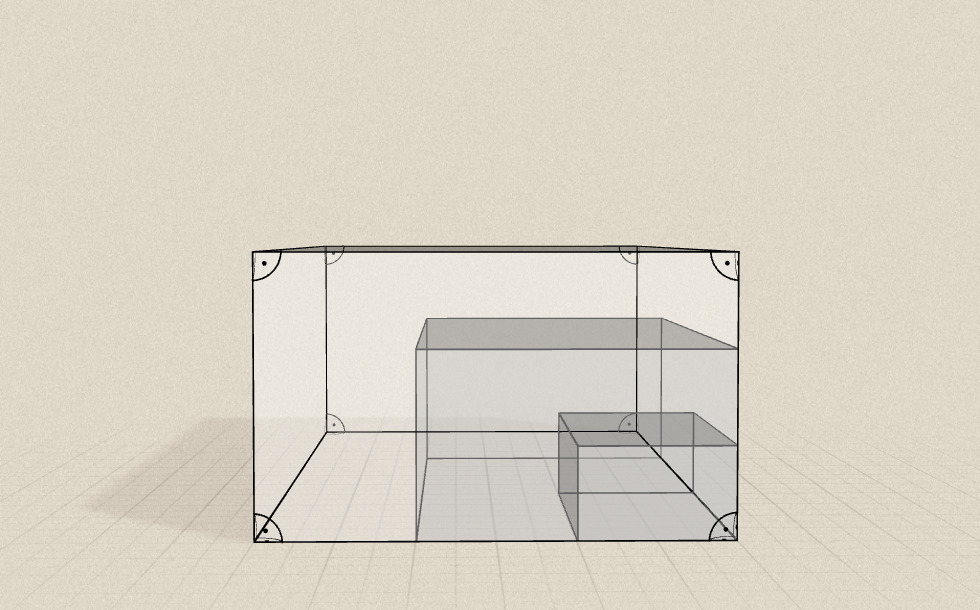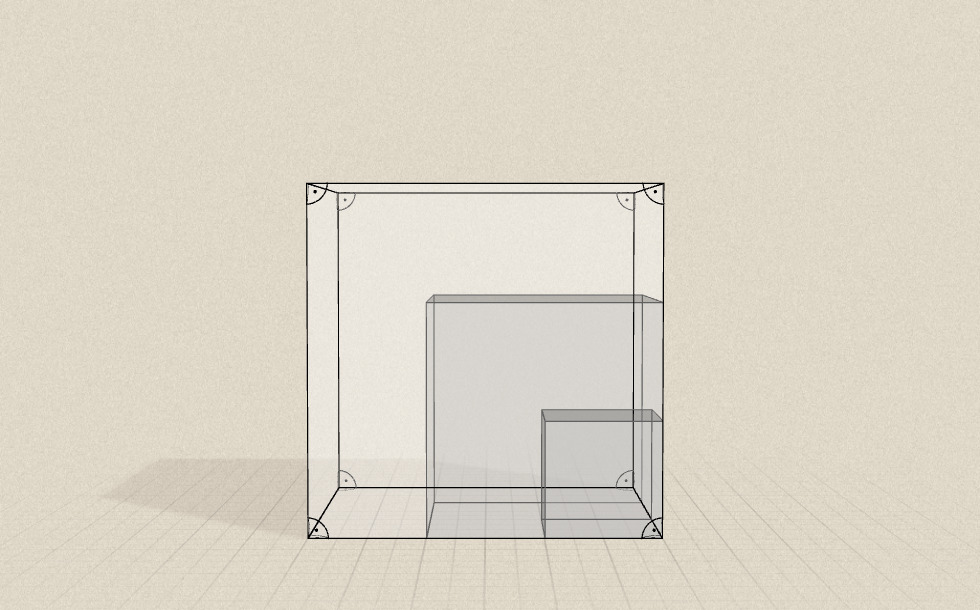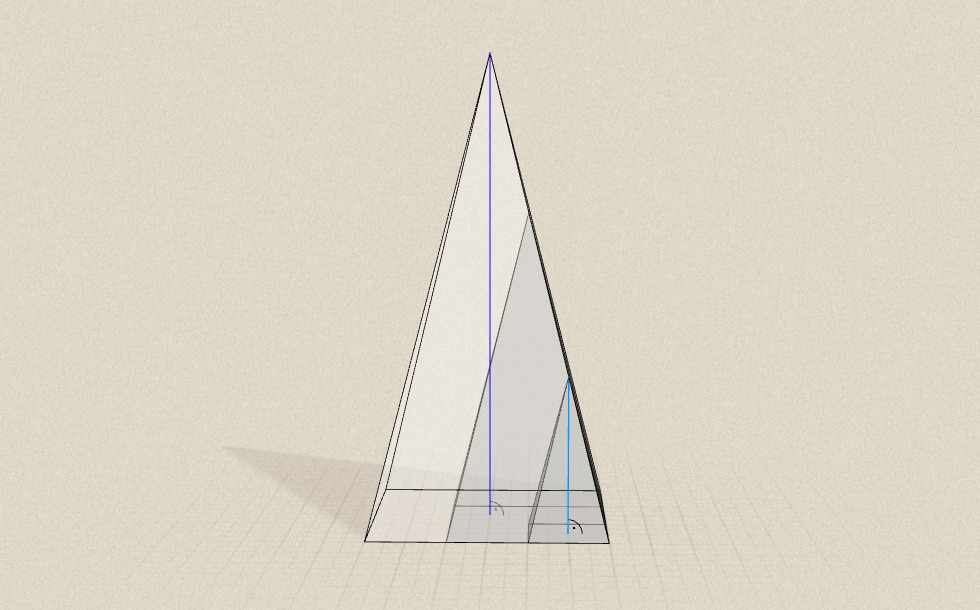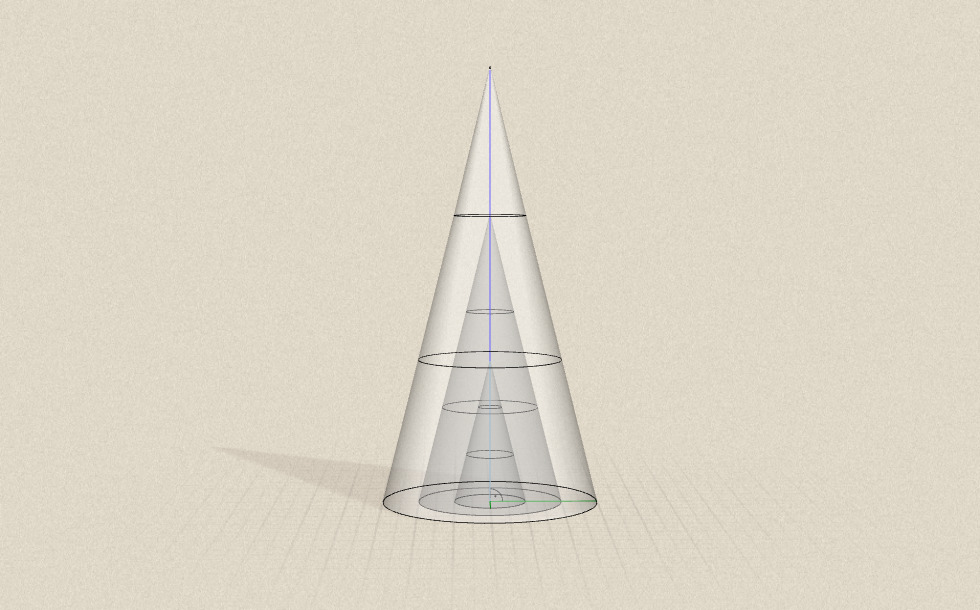A right circular cone is a cone with a circular base in which the orthogonal projection of the apex on the bottom base coincides with the center of the base. The volume of a right circular cone is one third the product of its base area (r²π) and its height (h).

If we enlarge a right circular cone by a scale factor of 2, both the radius of the base and the height will be doubled. Since both the base of the power and the other factor in the formula for the cone the volume are doubled, the volume of the enlarged right circular cone is 8 times larger than that of the original one.

If we enlarge a right circular cone by a scale factor λ of 3, both the radius of the base and the height will be tripled. Since both the base of the power and the other factor in the formula for the cone the volume are tripled, the volume of the enlarged right circular cone is 27 times larger than that of the original one.

In general, if we enlarge a right circular cone by a scale factor λ, its volume increases by λ³.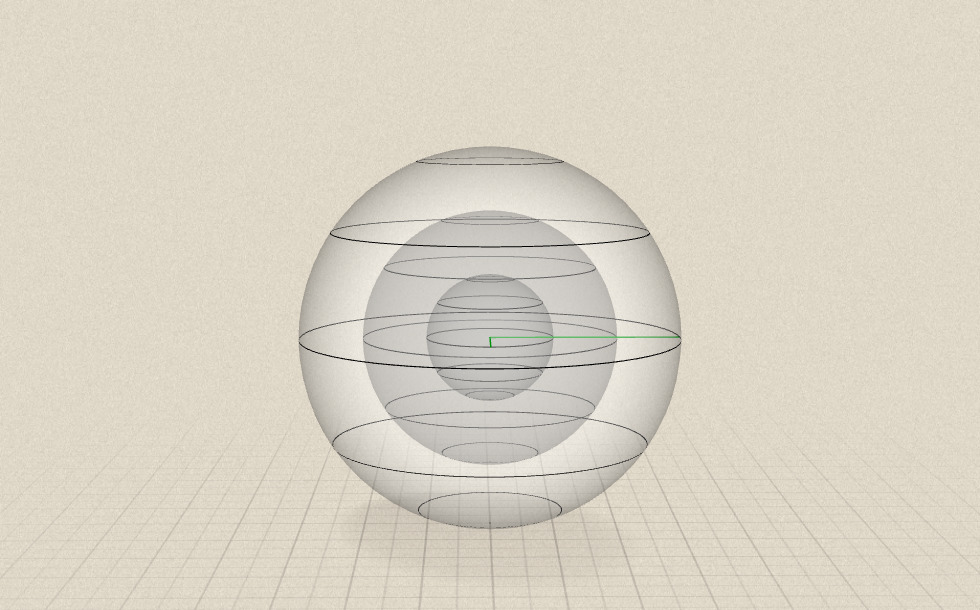A sphere is the set of points in space that are at equal distance from a given point in space (the center of the sphere, O). The volume of a sphere is equal to four-thirds the product of π and the cube of the radius of the sphere.

If we enlarge a sphere by a scale factor of 2, the length of its radius will be doubled. Since the base of the power in the formula for the volume of the sphere is doubled, so the volume of the enlarged sphere is 8 times larger than that of the original one.

If we enlarge a sphere by a scale factor λ of 3, the length of its radius will be tripled. Since the base of the power in the formula for the volume of the sphere is tripled, so the volume of the enlarged sphere is 27 times larger than that of the original one.

In general, if we enlarge a sphere by a scale factor λ, its volume increases by λ³.

### Related items#### Perimeter, area, surface area and volume

This animation presents the formulas to calculate the perimeter and area of shapes as well as the surface area and volume of solids.#### Volume of spheres (Cavalieri´s principle)

Calculating the volume of a sphere is possible using an appropriate cylinder and cone.#### Volume of spheres (demonstration)

The sum of the volume of the ´tetrahedrons´ gives an approximation of the volume of the sphere.#### Conic solids

This animation demonstrates various types of cones and pyramids.#### Cube

This animation demonstrates the components (vertices, edges, diagonals and faces) of the cube, one of the Platonic solids.#### Cuboid

A cuboid is a polyhedron with six rectangular faces.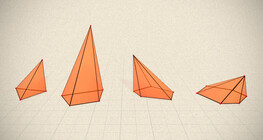#### Regular square pyramid

A regular square pyramid is a right pyramid with a square base and four triangular faces.#### Volume and surface area (exercise)

An exercise about the volume and surface area of solids generated from a ´base cube´.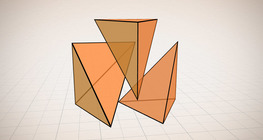#### Volume of a tetrahedron

To calculate the volume of a tetrahedron we start by calculating the volume of a prism.#### Grouping of cuboids

This animation demonstrates various types of cuboids through everyday objects.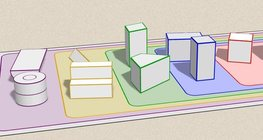#### Grouping of solids

This animation demonstrates various groups of solids through examples.#### Grouping of solids 1

This animation demonstrates various groups of solids through examples.#### Grouping of solids 2

This animation demonstrates various groups of solids through examples.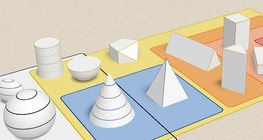#### Grouping of solids 3

This animation demonstrates various groups of solids through examples.#### Grouping of solids 4

This animation demonstrates various groups of solids through examples.